# :octopus: Generalized additive models in Python with a Bayesian twistmalmgrek, updated 🕥 2022-09-16 07:06:45

# Gammy – Generalized additive models in Python with a Bayesian twistA Generalized additive model is a predictive mathematical model defined as a sum of terms that are calibrated (fitted) with observation data.

Generalized additive models form a surprisingly general framework for building models for both production software and scientific research. This Python package offers tools for building the model terms as decompositions of various basis functions. It is possible to model the terms e.g. as Gaussian processes (with reduced dimensionality) of various kernels, as piecewise linear functions, and as B-splines, among others. Of course, very simple terms like lines and constants are also supported (these are just very simple basis functions).

The uncertainty in the weight parameter distributions is modeled using Bayesian statistical analysis with the help of the superb package BayesPy. Alternatively, it is possible to fit models using just NumPy.

## Installation

The package is found in PyPi.

```shell pip install gammy```

## Examples

In this overview, we demonstrate the package's most important features through common usage examples.

### Polynomial regression on 'roids

A typical simple (but sometimes non-trivial) modeling task is to estimate an unknown function from noisy data. First we import the bare minimum dependencies to be used in the below examples:

```python

import numpy as np

import gammy from gammy.models.bayespy import GAM

```

Let's simulate a dataset:

```python

np.random.seed(42)

n = 30 input_data = 10 * np.random.rand(n) y = 5 * input_data + 2.0 * input_data ** 2 + 7 + 10 * np.random.randn(n)

```

The object `x` is just a convenience tool for defining input data maps as if they were just Numpy arrays:

```python

from gammy.arraymapper import x

```

Define and fit the model:

```python

a = gammy.formulae.Scalar(prior=(0, 1e-6)) b = gammy.formulae.Scalar(prior=(0, 1e-6)) bias = gammy.formulae.Scalar(prior=(0, 1e-6)) formula = a * x + b * x ** 2 + bias model = GAM(formula).fit(input_data, y)

```

The model attribute `model.theta` characterizes the Gaussian posterior distribution of the model parameters vector.

``` python

model.mean_theta [array([3.20130444]), array([2.0420961]), array([11.93437195])]

```

Variance of additive zero-mean normally distributed noise is estimated automagically:

``` python

round(model.inv_mean_tau, 8) 74.51660744

```

#### Predicting with model

```python

model.predict(input_data[:2]) array([ 52.57112684, 226.9460579 ])

```

Predictions with uncertainty, that is, posterior predictive mean and variance can be calculated as follows:

```python

model.predict_variance(input_data[:2]) (array([ 52.57112684, 226.9460579 ]), array([79.35827362, 95.16358131]))

```

#### Plotting results

```python

fig = gammy.plot.validation_plot( ... model, ... input_data, ... y, ... grid_limits=[0, 10], ... input_maps=[x, x, x], ... titles=["a", "b", "bias"] ... )

```

The grey band in the top figure is two times the prediction standard deviation and, in the partial residual plots, two times the respective marginal posterior standard deviation.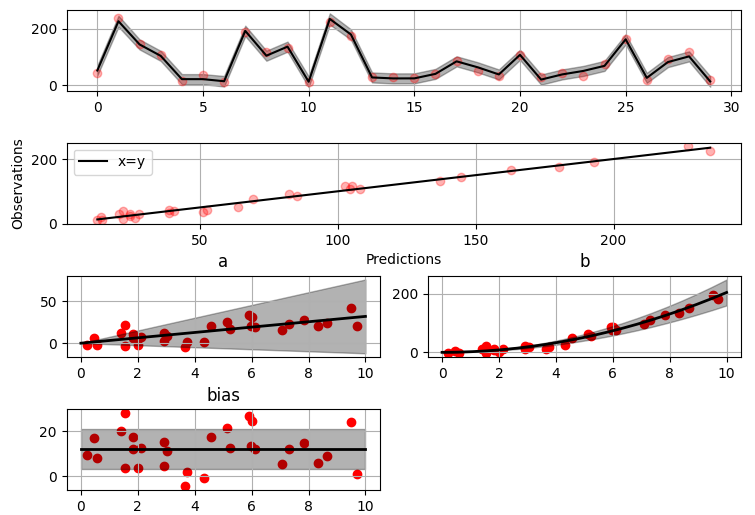It is also possible to plot the estimated Γ-distribution of the noise precision (inverse variance) as well as the 1-D Normal distributions of each individual model parameter.

Plot (prior or posterior) probability density functions of all model parameters:

```python

fig = gammy.plot.gaussian1d_density_plot(model)

```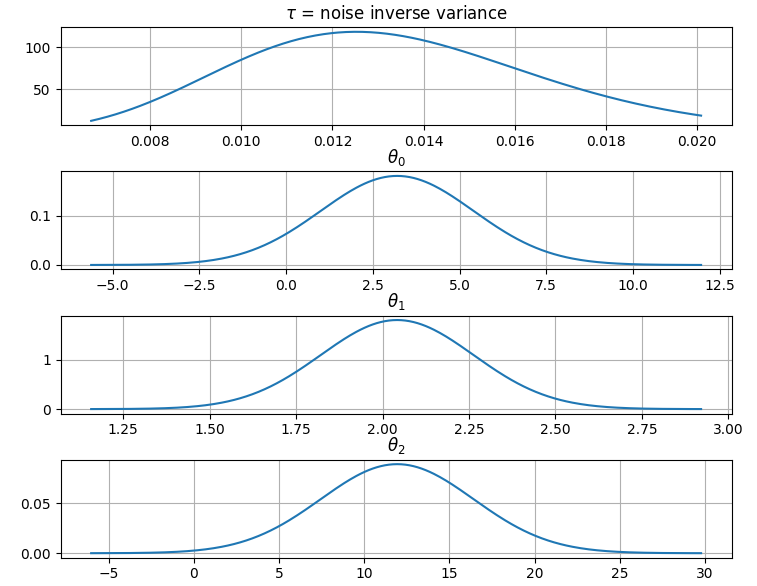#### Saving model on hard disk

Saving:

```python

model.save("/home/foobar/test.hdf5") ```

```python

### Gaussian process regression

Create fake dataset:

```python

n = 50 input_data = np.vstack((2 * np.pi * np.random.rand(n), np.random.rand(n))).T y = ( ... np.abs(np.cos(input_data[:, 0])) * input_data[:, 1] + ... 1 + 0.1 * np.random.randn(n) ... )

```

Define model:

``` python

a = gammy.formulae.ExpSineSquared1d( ... np.arange(0, 2 * np.pi, 0.1), ... corrlen=1.0, ... sigma=1.0, ... period=2 * np.pi, ... energy=0.99 ... ) bias = gammy.Scalar(prior=(0, 1e-6)) formula = a(x[:, 0]) * x[:, 1] + bias model = gammy.models.bayespy.GAM(formula).fit(input_data, y)

round(model.mean_theta, 8) -0.8343458

```

Plot predictions and partial residuals:

``` python

fig = gammy.plot.validation_plot( ... model, ... input_data, ... y, ... grid_limits=[[0, 2 * np.pi], [0, 1]], ... input_maps=[x[:, 0:2], x[:, 1]], ... titles=["Surface estimate", "intercept"] ... )

```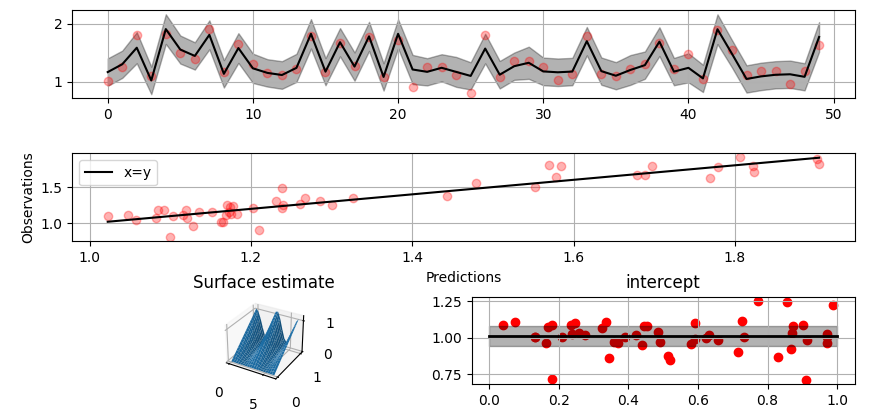Plot parameter probability density functions

``` python

fig = gammy.plot.gaussian1d_density_plot(model)

```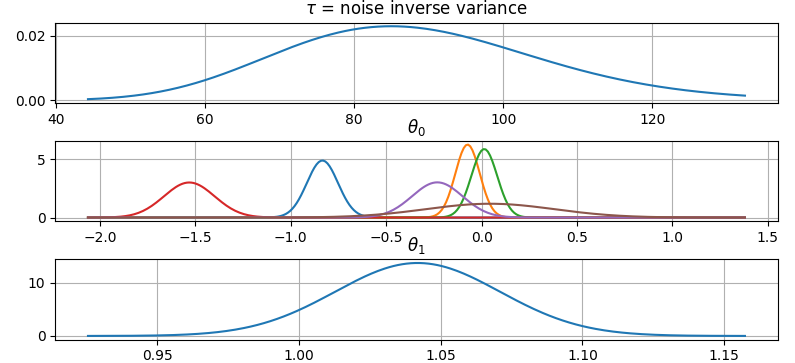#### More covariance kernels

The package contains covariance functions for many well-known options such as the Exponential squared, Periodic exponential squared, Rational quadratic, and the Ornstein-Uhlenbeck kernels. Please see the documentation section More on Gaussian Process kernels for a gallery of kernels.

### Spline regression

Constructing B-Spline based 1-D basis functions is also supported. Let's define dummy data:

```python

n = 30 input_data = 10 * np.random.rand(n) y = 2.0 * input_data ** 2 + 7 + 10 * np.random.randn(n)

```

Define model:

``` python

grid = np.arange(0, 11, 2.0) order = 2 N = len(grid) + order - 2 sigma = 10 ** 2 formula = gammy.BSpline1d( ... grid, ... order=order, ... prior=(np.zeros(N), np.identity(N) / sigma), ... extrapolate=True ... )(x) model = gammy.models.bayespy.GAM(formula).fit(input_data, y)

round(model.mean_theta, 8) -49.00019115

```

Plot validation figure:

``` python

fig = gammy.plot.validation_plot( ... model, ... input_data, ... y, ... grid_limits=[-2, 12], ... input_maps=[x], ... titles=["a"] ... )

```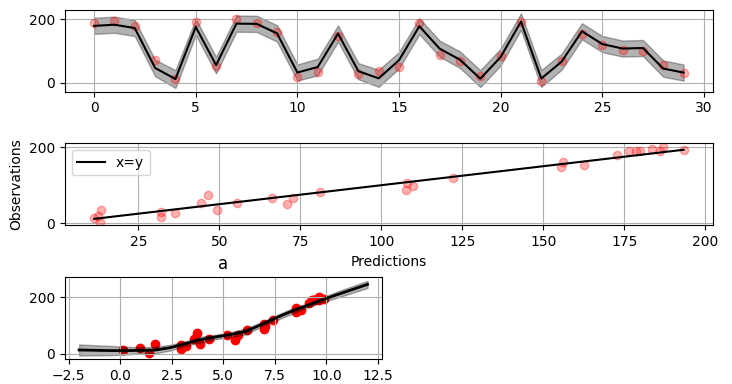Plot parameter probability densities:

``` python

fig = gammy.plot.gaussian1d_density_plot(model)

```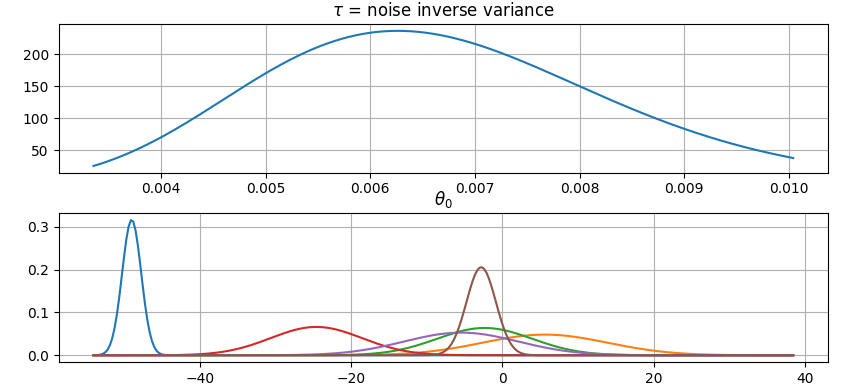### Non-linear manifold regression

In this example we try estimating the bivariate "MATLAB function" using a Gaussian process model with Kronecker tensor structure (see e.g. PyMC3). The main point in the below example is that it is quite straightforward to build models that can learn arbitrary 2D-surfaces.

Let us first create some artificial data using the MATLAB function!

```python

n = 100 input_data = np.vstack(( ... 6 * np.random.rand(n) - 3, 6 * np.random.rand(n) - 3 ... )).T y = ( ... gammy.utils.peaks(input_data[:, 0], input_data[:, 1]) + ... 4 + 0.3 * np.random.randn(n) ... )

```

There is support for forming two-dimensional basis functions given two one-dimensional formulas. The new combined basis is essentially the outer product of the given bases. The underlying weight prior distribution priors and covariances are constructed using the Kronecker product.

```python

a = gammy.ExpSquared1d( ... np.arange(-3, 3, 0.1), ... corrlen=0.5, ... sigma=4.0, ... energy=0.99 ... )(x[:, 0]) # NOTE: Input map is defined here! b = gammy.ExpSquared1d( ... np.arange(-3, 3, 0.1), ... corrlen=0.5, ... sigma=4.0, ... energy=0.99 ... )(x[:, 1]) # NOTE: Input map is defined here! A = gammy.formulae.Kron(a, b) bias = gammy.formulae.Scalar(prior=(0, 1e-6)) formula = A + bias model = GAM(formula).fit(input_data, y)

round(model.mean_theta, 8) 0.37426986

```

Note that same logic could be used to construct higher dimensional bases, that is, one could define a 3D-formula:

```python

formula_3d = gammy.Kron(gammy.Kron(a, b), c)

```

Plot predictions and partial residuals:

```python

fig = gammy.plot.validation_plot( ... model, ... input_data, ... y, ... grid_limits=[[-3, 3], [-3, 3]], ... input_maps=[x, x[:, 0]], ... titles=["Surface estimate", "intercept"] ... )

```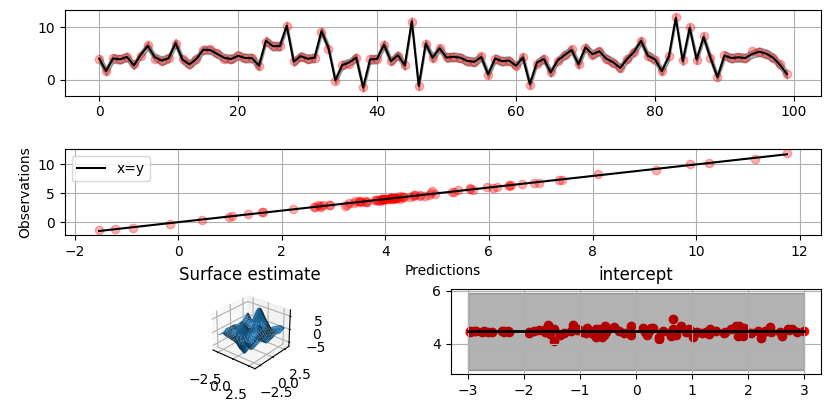Plot parameter probability density functions:

```

fig = gammy.plot.gaussian1d_density_plot(model)

```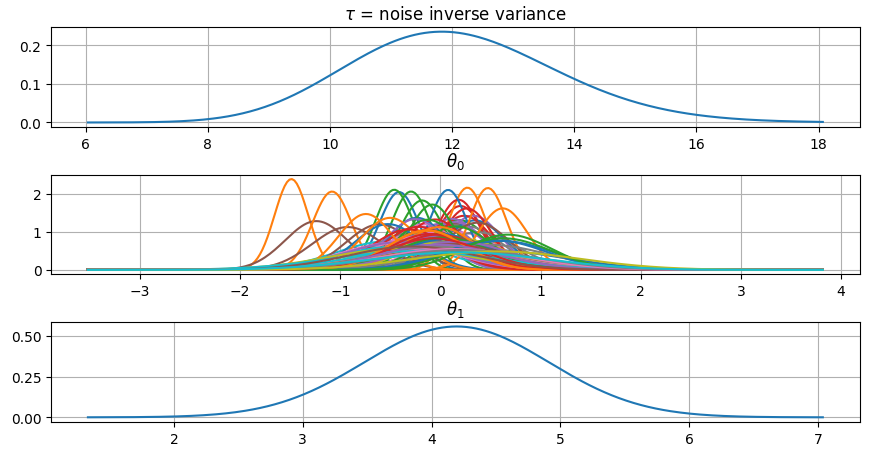## Testing

The package's unit tests can be ran with PyTest (`cd` to repository root):

```shell pytest -v```

Running this documentation as a Doctest:

```shell python -m doctest -v README.md```

## Package documentation

Documentation of the package with code examples: https://malmgrek.github.io/gammy.

## Issues

### Tweedie distribution

opened on 2021-06-01 15:14:00 by nickcorona

Is there a way to fit a gam with a Tweedie distribution?

A Tweedie distribution is a mixture between a poison and a gamma distribution. It helps model insurance losses with a significant positive probability mass at 0 (because most customers do not claim losses).

### WIP: Begin work on ExpSquaredAnalytic1d

opened on 2020-02-21 23:01:50 by kinnala

This is not yet ready but shows the basic idea. Here is one comparison between numerical (blue line) and analytic (orange line) basis: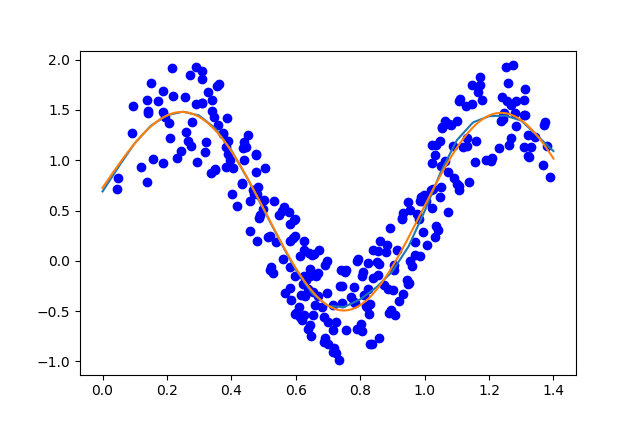#####malmgrek

Data scientist & software developer. Interested in math and coding.

bayesian-inference machine-learning statistics mathematical-modelling